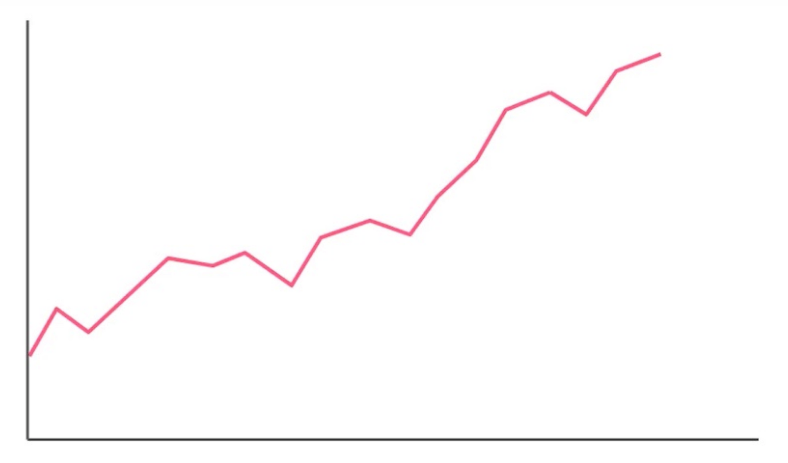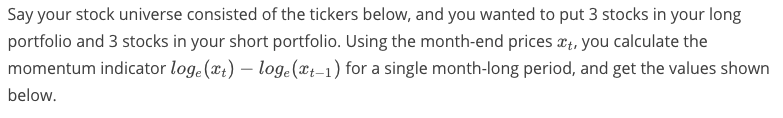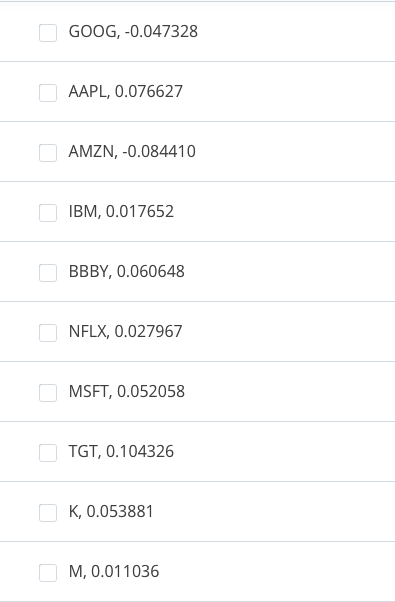A set of rules that determine what stocks to trade,when to trade,and how much money to inves.

## Momentum-based Signals

### Newton's First Law of Motion

An object at rest stays at rest and an object in motion stays in motion with the same speed and in the same direction unless acted upon by an unbalaced force.

### quize:Momentum-based SignalsConsider the above image. Assuming an investor thinks that the momentum effect applies to this stock during this time period, what does the investor think will occur?

A: the stock price will likely increase
B: the stock price will likely decrease

## Long and Short Positions

### Portfolio 投资组合

A portfolio is a collection of investments held and/or managed by an investment company, hedge fund, financial institution or individual.

### Long 长期

A long (or long position) is the purchase of an asset under the expectation that the price of the asset will rise.

### short 短期

A short (or short position) is the selling of an asset under the expectation that the price of the asset will decline. In practice, an investor profits from a short position by borrowing shares from a brokerage firm (agreeing to pay an interest rate as a fee), selling them on the open market, and later buying them back on the open market at a lower price and returning them to the brokerage firm.

Dtype

## Data Type Object

Let's look into how you might generate positions from signals. To do that, we first need to know about dtype or data type objects in Numpy.

A data type object is a class that represents the data. It's similar to a [data type](data type), but contains more information about the data. Let's see an example of a data type object in Numpy using the array array.

import numpy as np

array = np.arange(10)

print(array)
print(type(array))
print(array.dtype)
[0 1 2 3 4 5 6 7 8 9]
<class 'numpy.ndarray'>
int64

From this, we see array is a numpy.ndarray with the data [0 1 2 3 4 5 6 7 8 9] represented as int64 (64-bit integer).

Let's see what happens when we divide the data by 2 to generate not integer data.

float_arr = array / 2

print(float_arr)
print(type(float_arr))
print(float_arr.dtype)
[ 0.   0.5  1.   1.5  2.   2.5  3.   3.5  4.   4.5]
<class 'numpy.ndarray'>
float64

The array returned has the values [ 0. 0.5 1. 1.5 2. 2.5 3. 3.5 4. 4.5], which is what you would expect for divinding by 2. However, since this data can't be represeted by integers, the array is now represented as float64 (64-bit float).

How would we convert this back to int64? We'll use the ndarray.astype function to cast it from it's current type to the type of int64 (np.int64).

int_arr = float_arr.astype(np.int64)

print(int_arr)
print(type(int_arr))
print(int_arr.dtype)
[0 0 1 1 2 2 3 3 4 4]
<class 'numpy.ndarray'>
int64

This casts the data to int64, but all also changes the data. Since fractions can't be represented as integers, the decimal place is dropped.

## Signals to Positions

Now that you've seen how the a data type object is used in Numpy, let's see how to use it to generate positions from signals. Let's use prices array to represent the prices in dollars over time for a single stock.

prices = np.array([1, 3, -2, 9, 5, 7, 2])

prices
array([ 1,  3, -2,  9,  5,  7,  2])

For the positions, let's say we want to buy one share of stock when the price is above 2 dollars and the buy 3 more shares when it's above 4 dollars. We'll first need to generate the signal for these two positions.

signal_one = prices > 2
signal_three = prices > 4

print(signal_one)
print(signal_three)
[False  True False  True  True  True False]
[False False False  True  True  True False]

This gives us the points in time for the signals above 2 dollars and above 4 dollars. To turn this into positions, we need to multiply each array by the respective amount to invest. We first need to turn each signal into an integer using the ndarray.astype function.

signal_one = signal_one.astype(np.int)
signal_three = signal_three.astype(np.int)

print(signal_one)
print(signal_three)
[0 1 0 1 1 1 0]
[0 0 0 1 1 1 0]

Now we multiply each array by the respective amount to invest.

pos_one = 1 * signal_one
pos_three = 3 * signal_three

print(pos_one)
print(pos_three)
[0 1 0 1 1 1 0]
[0 0 0 3 3 3 0]

If we add them together, we have the final position of the stock over time.

long_pos = pos_one + pos_three

print(long_pos)
[0 1 0 4 4 4 0]

## Quiz

Using this information, implement generate_positions using Pandas's df.astype function to convert prices to final positions using the following signals:

• Long 30 share of stock when the price is above 50 dollars
• Short 10 shares of stock when it's below 20 dollars
import project_tests

def generate_positions(prices):
"""
Generate the following signals:
- Long 30 share of stock when the price is above 50 dollars
- Short 10 shares when it's below 20 dollars

Parameters
----------
prices : DataFrame
Prices for each ticker and date

Returns
-------
final_positions : DataFrame
Final positions for each ticker and date
"""
# TODO: Implement Function
signal_30 = prices > 50
signal_10 = prices < 20

signal_30 = signal_30.astype(np.int)
signal_10 = signal_10.astype(np.int)

signal_30 = signal_30 * 30
signal_10 = signal_10 * (-10)

long_pos = signal_30 + signal_10

return long_pos

project_tests.test_generate_positions(generate_positions)
Tests Passed

## Combining Long-Short (Clarification)

Note that the example in the video had some simplifying assumptions, where each stock has the same number of dollars invested, so that the portfolio weights for each stock are the same.

It also includes the simplifying assumption that both the long and short portfolio have the same dollar amount invested (in terms of absolute magnitude), in which case the combination of the long and short portfolios would also be the simple average between the two. To correct the video, this should be (long + short) / 2; where the short is a negative value.

## Quize:Momentum-based Portfolio

### Short PortfolioWhen we build a portfolio of stocks to short based on the idea of profiting from the momentum effect, we look for poor performers, which we think will continue to perform badly. Which 3 stocks would you short for this month-long period?## Quize:

Top and Bottom Performing

Let's look at how we might get the top performing stocks for a single period. For this example, we'll look at just a single month of closing prices:

import pandas as pd

month = pd.to_datetime('02/01/2018')
close_month = pd.DataFrame(
{
'A': 1,
'B': 12,
'C': 35,
'D': 3,
'E': 79,
'F': 2,
'G': 15,
'H': 59},
[month])

close_month
A B C D E F G H
2018-02-01 1 12 35 3 79 2 15 59

close_month gives use the prices for the month of February, 2018 for all the stocks in this universe (A, B, C, ...). Looking at these prices, we can see that the top 2 performing stocks for that month was E and H with the prices 79 and 59.

To get this using code, we can use the Series.nlargest function. This function returns the items with the n largest numbers. For the example we just talked about, our n is 2.

try:
# Attempt to run nlargest
close_month.nlargest(2)
except TypeError as err:
print('Error: {}'.format(err))

What happeened here? It turns out we're not calling the Series.nlargest function, we're actually calling DataFrame.nlargest, since close_month is a DataFrame. Let's get the Series from the dataframe using .loc[month], where month is the 2018-02-01 index created above.

close_month.loc[month].nlargest(2)
E    79
H    59
Name: 2018-02-01 00:00:00, dtype: int64

Perfect! That gives us the top performing tickers for that month. Now, how do we get the bottom performing tickers? There's two ways to do this. You can use Panda's Series.nsmallest function or just flip the sign on the prices and then apply DataFrame.nlargest. Either way is fine. For this course, we'll flip the sign with nlargest. This allows us to reuse any funtion created with nlargest to get the smallest.

To get the bottom 2 performing tickers from close_month, we'll flip the sign.

(-1 * close_month).loc[month].nlargest(2)
A   -1
F   -2
Name: 2018-02-01 00:00:00, dtype: int64

That gives us the bottom performing tickers, but not the actual prices. To get this, we can flip the sign from the output of nlargest.

(-1 * close_month).loc[month].nlargest(2) *-1
A    1
F    2
Name: 2018-02-01 00:00:00, dtype: int64

Now you've seen how to get the top and bottom performing prices in a single month. Let's see if you can apply this knowledge.

## Quiz:Top and Bottom Performing

Implement date_top_industries to find the top performing closing prices and return their sectors for a single date. The function should only return the set of sectors, there shouldn't be any duplicates returned.

• The number of top performing prices to look at is represented by the parameter top_n.
• The date parameter is the date to look for the top performing prices in the prices DataFrame.
• The sector information for each ticker is located in the sector parameter.

For example:

                 Prices
A         B         C         D         E
2013-07-08     2         2         7         2         6
2013-07-09     5         3         6         7         5
...            ...       ...       ...

Sector
A       "Utilities"
B       "Health Care"
C       "Real Estate"
D       "Real Estate"
E       "Information Technology"

Date:  2013-07-09
Top N: 3

The set created from the function date_top_industries should be the following:

{"Utilities", "Real Estate"}

Note: Stock A and E have the same price for the date, but only A's sector got returned. We'll keep it simple and only take the first occurrences of ties.

import project_tests

def date_top_industries(prices, sector, date, top_n):
"""
Get the set of the top industries for the date

Parameters
----------
prices : DataFrame
Prices for each ticker and date
sector : Series
Sector name for each ticker
date : Date
Date to get the top performers
top_n : int
Number of top performers to get

Returns
-------
top_industries : set
Top industries for the date
"""
# TODO: Implement

print("Print prices\n",prices)
print()
print("Print sector\n", sector)
print()
print(prices.loc[date].nlargest(top_n).index)

print()
res = set(sector.loc[prices.loc[date].nlargest(top_n).index])
print("Print res:\n", res)

return set(sector.loc[prices.loc[date].nlargest(top_n).index])

project_tests.test_date_top_industries(date_top_industries)
Print prices
DNF         TYR         NBD         EBIQ        VDJS  \
2011-10-28 21.05081048 17.01384381 10.98450376  11.24809343 12.96171273
2011-10-29 15.63570259 14.69054309 11.35302769 475.74195118 11.95964043

KDRA        TSME           CUZ         TSD        FIVU
2011-10-28 482.34539247 35.20258059 3516.54167823 66.40531433 13.50396048
2011-10-29  10.91893302 17.90864387   24.80126542 12.48895419 15.63570259

Print sector
DNF          ENERGY
TYR       MATERIALS
NBD          ENERGY
EBIQ         ENERGY
VDJS        TELECOM
KDRA     FINANCIALS
TSME     TECHNOLOGY
CUZ          HEALTH
TSD       MATERIALS
FIVU    REAL ESTATE
dtype: object

Index(['EBIQ', 'CUZ', 'TSME', 'DNF'], dtype='object')

Print res:
{'ENERGY', 'HEALTH', 'TECHNOLOGY'}
Tests Passed